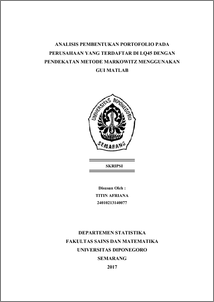# ANALISIS PEMBENTUKAN PORTOFOLIO PADA PERUSAHAAN YANG TERDAFTAR DI LQ45 DENGAN PENDEKATAN METODE MARKOWITZ MENGGUNAKAN GUI MATLAB

AFRIANA, TITIN (2017) ANALISIS PEMBENTUKAN PORTOFOLIO PADA PERUSAHAAN YANG TERDAFTAR DI LQ45 DENGAN PENDEKATAN METODE MARKOWITZ MENGGUNAKAN GUI MATLAB. Undergraduate thesis, Fakultas Sains dan Matematika, Undip.Preview
PDF
3388Kb

## Abstract

Portfolio is one of ways in investment activity that undertaken by more than one asset with intent to determining the amount of proportion of investment that to be made in a certain period of time. To determine the maximum result from making a portofolio, one of analysis model which can be used is markowitz. Markowitz exressed through diversification concept (with making of the optimal stock of portfolio), investor can maximize the expected income from investments with specific risk level or seeking to minimize risk to target certain profit level. To simplify the calculation of the portfolio for public, there is an application that made by using GUI in Matlab. Matlab (Matrix Laboratory) is an interactive programming system with basic elements of array database which dimensions do not need to be stated in a particular way, while the GUI is the submenu of Matlab. Generally, Matlab GUI is more easily learned and used because it worked without need to know the commandments and how the command works. The data used in this study consists of five types of assets in the LQ45 group, there are BBNI, PWON, PTBA, INCO, dan KLBF. In determining the portfolio proportion used trial and error method and Lagrange method. Based on the portfolio proportion of both methods obtained the optimal portfolio is almost the same. Keywords: GUI Matlab, LQ45, Portfolio, Markowitz, Trial and Error, Lagrange

Item Type: Thesis (Undergraduate) H Social Sciences > HA Statistics Faculty of Science and Mathematics > Department of Statistics 55090 Mr Hasbi Yasin 26 Jul 2017 13:25 26 Jul 2017 13:25

Repository Staff Only: item control page